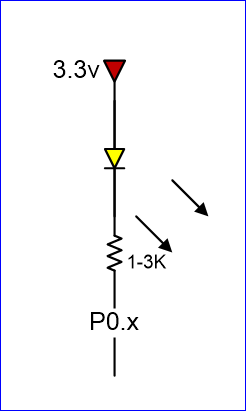# Difference between revisions of "Embedded System Tutorial GPIO"

## Objective

Interface your LPC17xx to a switch and an LED.

Although the interface may seem simple, you do need to consider hardware design and know some of the fundamental of electricity. There are a couple of goals for us :

• No hardware damage if faulty software is written.
• Circuit should prevent excess amount of current to avoid processor damage.

## Required Background

• You should know how to bit-mask variables
• You should know how to wire-wrap
• You should know fundamentals of electricity and the famous V = IR equation.

## GPIO

 GPIO stands for "General Purpose Input Output". Each pin can at least be used as an output or input. In an output configuration, the pin voltage is either 0v or 3.3v. In input mode, we can read whether the voltage is 0v or 3.3v.

## Coding

### Hardware Registers

The hardware registers map to physical pins. If we want to attach our switch and the LED to our microcontroller's PORT0, then here are the relevant registers and their functionality :

 LPC_GPIO0->FIODIR Direction of the port pins, 1 = output LPC_GPIO0->FIOPIN ```Read : Sensed inputs of the port pins, 1 = HIGH Write : Control voltage level of the pin, 1 = 3.3v ``` LPC_GPIO0->FIOSET Write only : Any bits written 1 are OR'd with FIOPIN LPC_GPIO0->FIOCLR Write only : Any bits written 1 are AND'd with FIOPIN

### Switch

 ```/* Make direction of PORT0.2 as input */ LPC_GPIO0->FIODIR &= ~(1 << 2); /* Now, simply read the 32-bit FIOPIN registers, which corresponds to * 32 physical pins of PORT0. We use AND logic to test if JUST the * pin number 2 is set */ if (LPC_GPIO0->FIOPIN & (1 << 2)) { // Switch is logical HIGH } else { // Switch is logical LOW }``` Note that the "inline" resistor is used such that if your GPIO is mis-configured as an OUTPUT pin, hardware damage will not occur from badly written software.

### LED

 ```/* Make direction of PORT0.3 as OUTPUT */ LPC_GPIO0->FIODIR |= (1 << 3); /* Setting bit 3 to 1 of IOPIN will turn ON LED * and resetting to 0 will turn OFF LED. */ LPC_GPIO0->FIOPIN |= (1 << 3); /* Faster, better way to set bit 3 (no OR logic needed) */ LPC_GPIO0->FIOSET = (1 << 3); /* Likewise, reset to 0 */ LPC_GPIO0->FIOCLR = (1 << 3);``` Given below are two configurations of an LED. Usually, the "sink" current is higher than "source", hence the active-low configuration is used more often.Active-low LED circuitActive-high LED circuit

## Conclusion

At the end of this lab, you should be familiar with how a microcontroller's memory can access physical pins.